Next: Second-order perturbation results Up: Theoretical models Previous: Equidistant-level model (infinitely many

## Deformed shell (finite non-degenerate spectrum)

In this section, we investigate the deformed-shell-plus-pairing Hamiltonian that contains the single-particle term (17) (for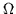twofold degenerate single-particle states available for the pair scattering) and seniority-pairing interaction (14). In this case, the analytic solution does not exist, but the exact eigenstates can be found numerically using, for example, the Richardson method  or by performing a direct diagonalization. The latter approach cannot be applied when the number of single-particle levels is large, because the dimension of the Hilbert space grows as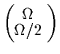, and this puts the practical limit at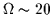. imaginary-time method

A particular variant of this model is when the single-particle spectrum is uniformly spaced (finite-number equidistant-level model), i.e., ek=kd (k=1,...,). In this work, we are mainly interested in the ability of the energy-spacing filter (7) to extract the single-particle spectrum from the total binding energies. Therefore, below, we also study the case of a nearly-equidistant spectrum, in which ek=kd except for the seventh level shifted up in energy by d/4 (i.e, e7=7.25 d). The value of the single-particle spacing d constitutes a convenient energy scale, and below, the results will be expressed as ratios of all energies and parameters with respect to d.

Figures 4 and 5 show the results of the exact calculations for the nearly-equidistant spectrum of=16 levels. The behavior of the three-mass filter (1) is illustrated in Fig. 4, where values of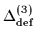are compared to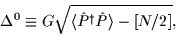(56)

which is the equivalent" pairing gap. (In the BCS limit,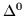becomes the gap parameter.)

For G/d=0.1 (weak pairing), values of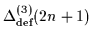nicely follow the low values of, while those of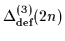are clearly influenced by the single-particle spectrum and do not at all reflect the smallness of the pairing correlations. In particular, the fluctuation around N=14 (n=7) clearly shows up inand is absent in.

In the case of intermediate pairing (G/d=0.3),behaves rather smoothly, whilezigzags in the region of irregularity in the spectrum. No direct correspondence between the values ofandcan be found here. However, with increasing values of G, i.e., when the static pairing sets in,closely approaches. This is nicely illustrated for G/d=0.5. Only in the case of relatively strong pairing correlations (but still far from the strong pairing limit discussed in Sec. 3.1.2) is the fluctuation inbarely visible.

In the case of weak pairing correlations, the corresponding energy-spacing filters (7), shown in Fig. 5, very well reflect the structure of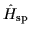. With an increasing pairing strength, the pairing Hamiltonian gives rise to a diffused Fermi surface (i.e., it smears out the single-particle occupations). Consequently, the information about the details of the single-particle distribution is then expected to be washed out. This is clearly seen in Fig. 5, where the nearly-equidistant single-particle spacings are marked by dots.

However, it is seen that even in the case of relatively strong pairing correlations, (G/d=0.4), the symmetric energy-spacing indicator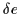gives a qualitative description of the spectrum. Even at G/d=0.5, the zigzag inappears in the right place. As far as the asymmetric filters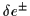are concerned, their behavior is more strongly influenced by G, and the resulting particle number dependence may make them less useful measures of the spectral properties.

In order to assess the quality of the quasi-particle (BCS) approximation for the binding-energy indicators, in the same model we have also carried out the BCS calculations. As seen in Fig. 6, it is only at large values of G that the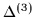filters (1) which are applied to the BCS energies approach the exact results. Especially at intermediate values of pairing strength, where the static pairing vanishes in odd-N nuclei, BCS becomes a rather poor approximation. However, it is clear that values of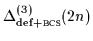are affected by the single-particle spectrum, while those of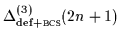are rather insensitive to it.

The agreement is significantly better for the energy-spacing filter(Fig. 7). Again, as discussed above, at large values of pairing strength,is only a qualitative measure of the single-particle splitting.Next: Second-order perturbation results Up: Theoretical models Previous: Equidistant-level model (infinitely many
Jacek Dobaczewski
2000-03-09